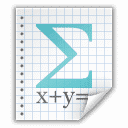Our Math Freebies

LOGIC

Propositional Logic, First-Order Logic, Proof Theory, Model Theory and the like!Axiom Schemes of Machover's PropCal

A 2-page handout on the 5 axiom schemes of PropCal (as defined in Moshé Machover's Set Theory, Logic and Their Limitations).First-Order Logic in Standard Notation — Basics

A 8-page meta-theoretical introduction to the language and semantics of first-order logic, as presented in modern standard notation.Bell & Machover's PropCal and its Structural Rules

A 9-oage primer on the proof system PropCal (as presented in Bell & Machover's A Course in Mathematical Logic), along with the 12 associated structural rules.

STATISTICS

Hypothesis Testing, Confidence Interval, Descriptive Statistics, Bayesian Inference and the like!Biostatistics — Review Sheets with Formulas

Originally developed for McGill EPIB 507 (Biostats for Health Science), this 5-page review sheet cover — in bullet-point form — a wide range of topics and formulas from descriptive statistics, probability distributions, hypothesis testing, confidence interval, regression and non-parametric methods.